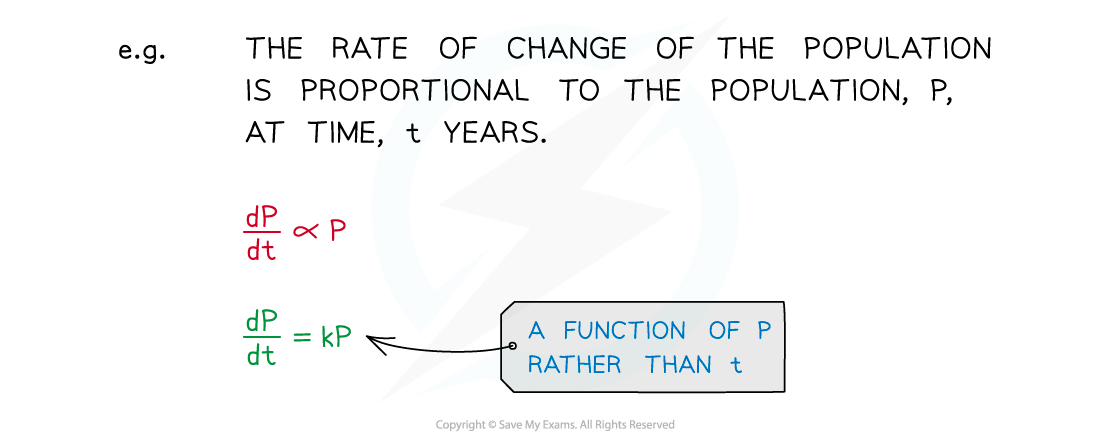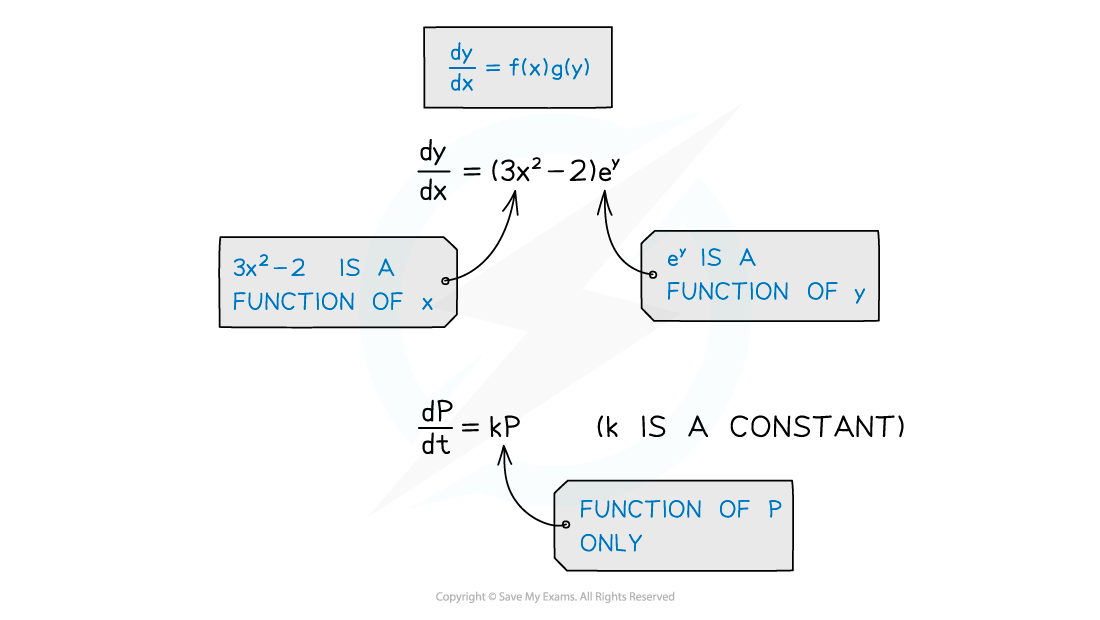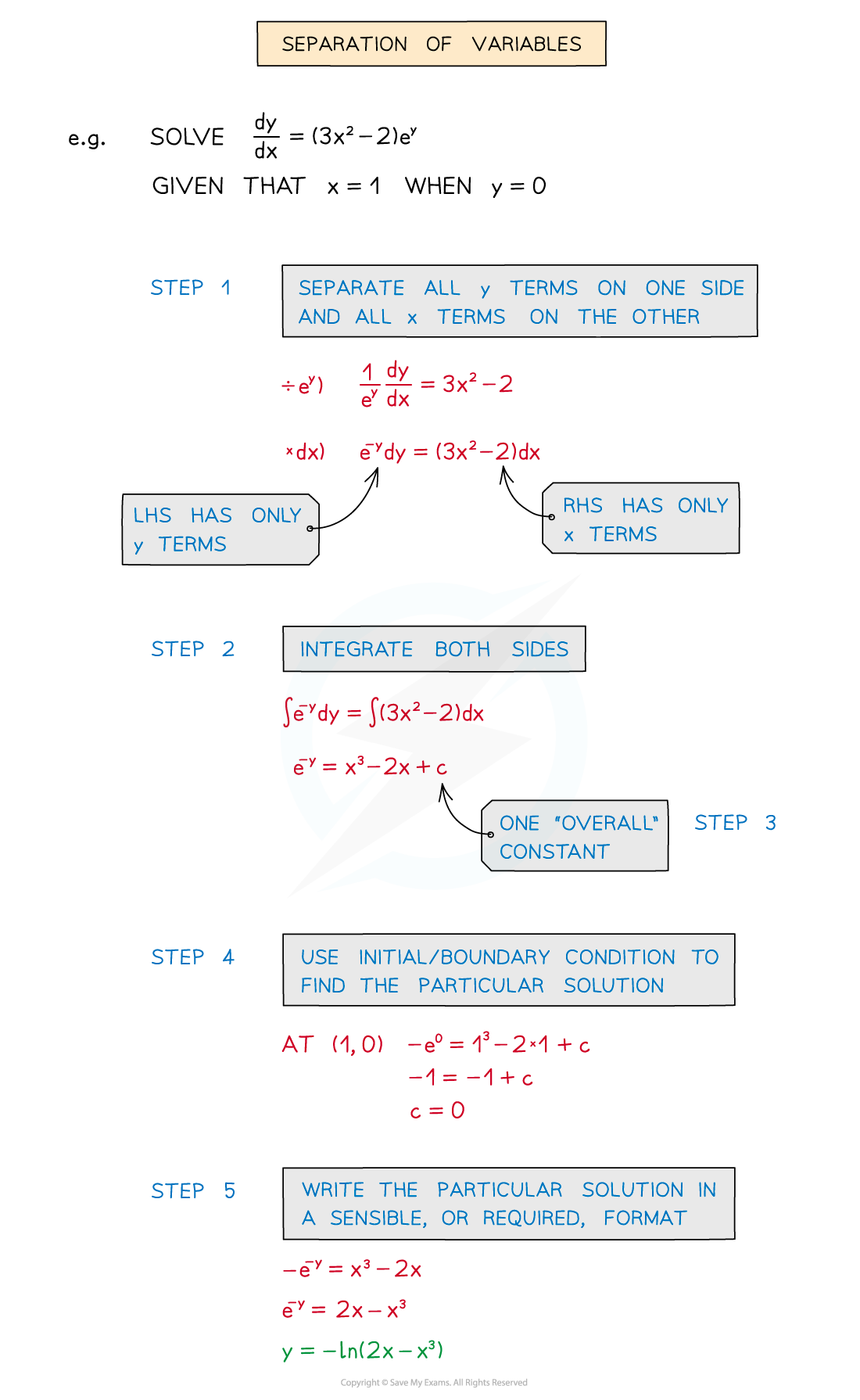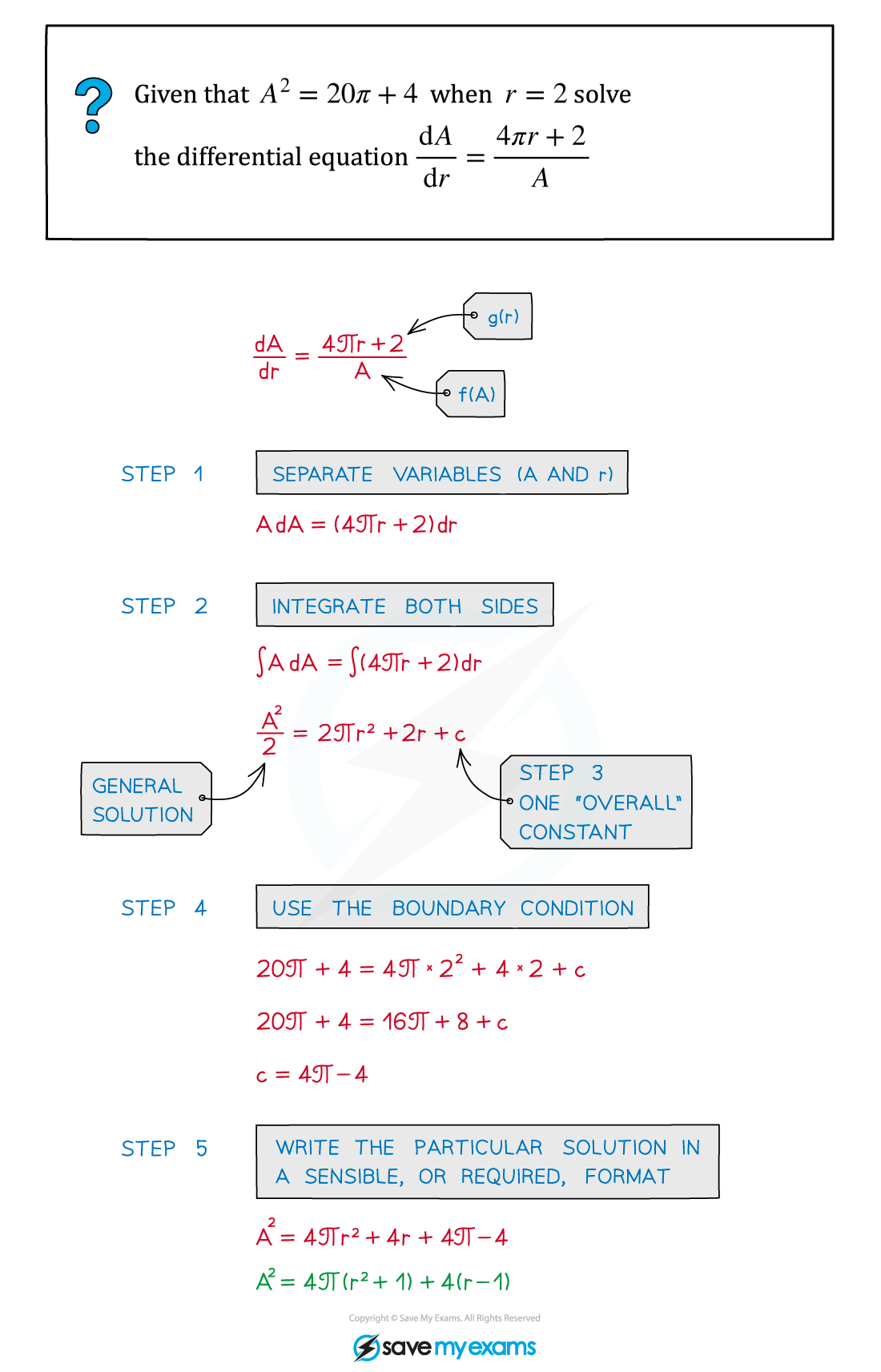# AQA A Level Maths: Pure复习笔记8.3.3 Separation of Variables

### Separation of Variables

#### What does separation of variables mean?• Many differential equations used in modelling either …
• … have two variables involved (ie x and y), or,
• ... involve a function of the dependent variable (ie y) only
• This is particularly true where proportionality is involved
• eg population change is dependent on both time and the size of the population• This type of question is covered in more detail in Modelling with Differential Equations

#### How do I know if I need to separate the variable in a question?• There is a product of functions in different variables
• ie dy/dx = f(x) × g(y)
• It will not be possible to integrate directly from an equation in the form dy/dx= g(y)

#### How do I solve a separating variables question?• STEP 1: Separate all y terms on one side and all x terms on the other side
• STEP 2: Integrate both sides
• STEP 3: Include one “overall” constant of integration
• STEP 4: Use the initial or boundary condition to find the particular solution
• STEP 5: Write the particular solution in sensible, or required, format

#### Worked Example